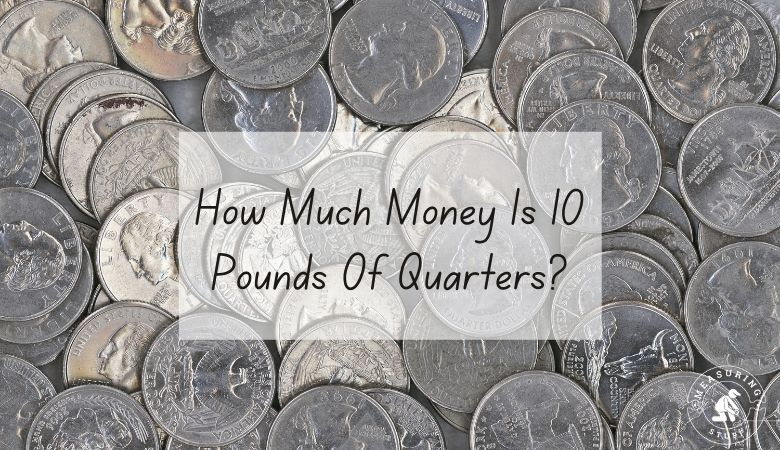# How Much Money Is 10 Pounds Of Quarters?

Last updated on May 8th, 2023 at 11:16 am

This article will show you exactly how much money you will have with 10 pounds of quarters.

If you are lucky enough to have 10 pounds of quarters, you may be wondering how much it is worth.

If you are able to weigh your quarters accurately on a scale, remember to subtract the weight of the container or bag that the coins are in.

So, how much money do you have with 10 pounds of quarters?

The answer is you will have \$200. A single quarter coin or twenty-five cent piece from the USA weighs 5.670 grams each which is 0.0125 pounds. Therefore 10 quarters weigh 0.125 pounds, 100 quarters weigh 1.25 pounds, 500 quarters weigh 6.25 pounds, 700 quarters weigh 8.75 pounds, and 800 quarters weigh 10 pounds.

To determine how much money is 10 pounds of quarters, you just need to use a simple formula.

# of pounds in coins/weight per coin = # of coins.

In the example above, 10 pounds / 0.0125 = 800.

Once you know how many coins you have, multiply that number by the value of the coin to tell you how much money you have.

800 quarters @ 0.25 cents each = \$200.

800 x 0.25 = 200.

Now you know that if you have a 10-pound bag of quarters, you have exactly \$200.

## How much does a thousand dollars in quarters weigh?

\$1000 in quarters weighs about 50 pounds which is 22,680 grams or 22.68 kilograms.